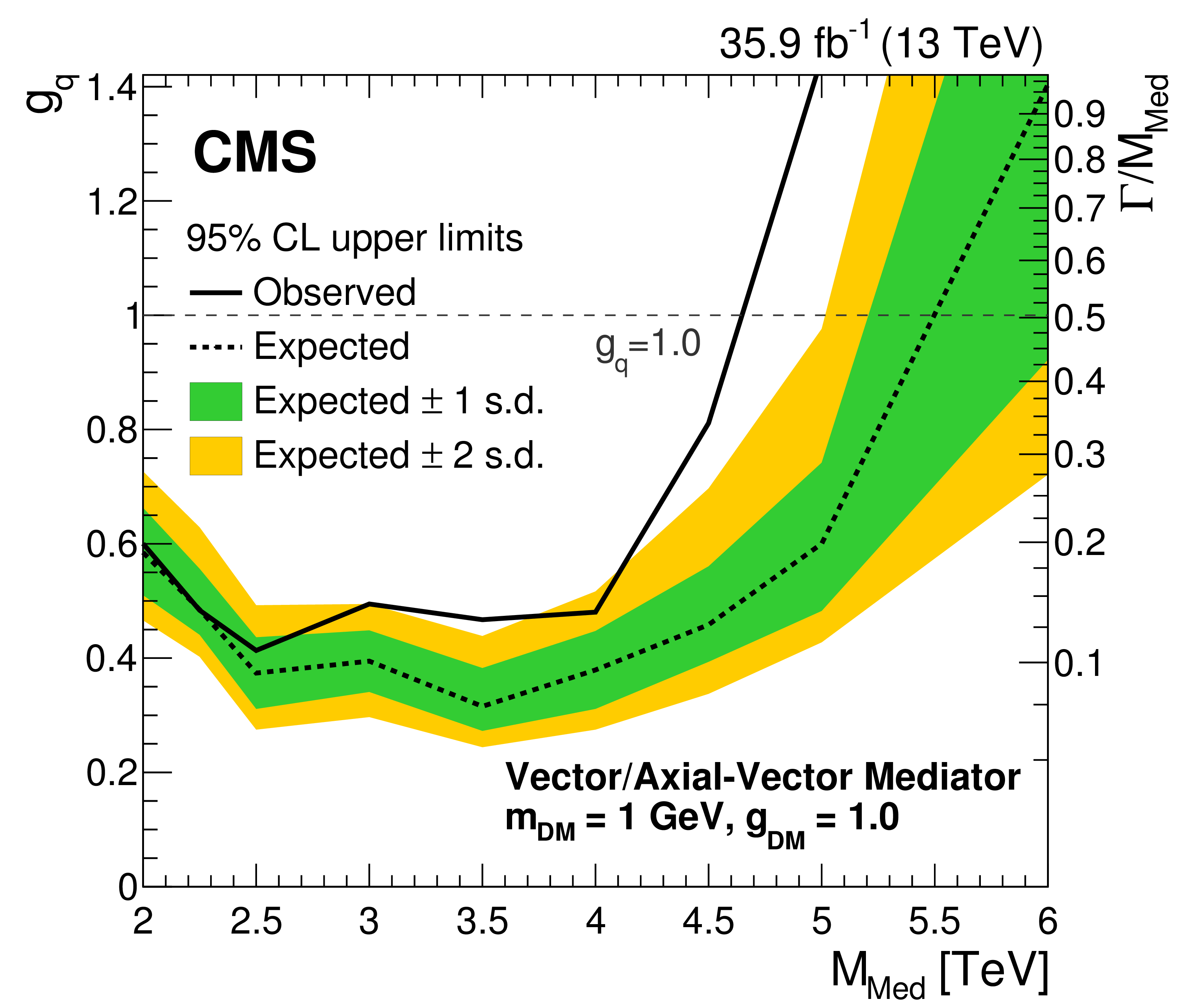## Thursday, October 25, 2018

### A 2.8-sigma CMS hint of a dark matter mediator

Even small deviations from the Standard Model – at the LHC and elsewhere – remain rare so we must discuss as weak ones as those whose statistical significance is just 2.8 sigma.Tommaso Dorigo has discussed various other channels and possibilities in which his CMS team found nothing. But let us focus on the pretty graph above. As you can see, the observed black curve jumps above the predicted green-and-yellow (1-and-2-sigma "Brazilian") band which means the excess of events that "look like" a certain signal.

The process showing this excess was taken from this March 2018 CMS paper, Figure 3 on Page 13/38. One assumes that one colliding proton contributes the quark $$q$$, the other one contributes the antiquark $$\bar q$$, and those merge into a new vector particle:$q\bar q \to V \to \dots$ Now, this vector particle, if the signal above were real, would probably be heavier than $$4.5\TeV$$ and it would be unstable and decay to$q\bar q \to V \to \chi\bar\chi$ where $$\chi$$ is my local symbol for the dark matter particle – in the most motivated cases, it is identical with its antiparticle $$\bar\chi$$ but for these interactions to exist, one could actually prefer $$\chi,\bar\chi$$ to be distinct (and carry a new charge).

I just described a process in the $$s$$-channel, one that has a new intermediate particle. But once this new particle and field exists, there also exist processes in the $$t$$- and $$u$$-channels which look like$q\chi \to q\chi V \to q\chi$ So the quark and the dark matter particle enter, one of them emits a virtual vector boson $$V$$, this gets absorbed by the other particle, and you're left with $$q$$ and $$\chi$$ again, but with different momenta. I just described some kind of an elastic collision between quarks (in practical experiments, nuclei) and dark matter particles, mediated by $$V$$.

The minimally interacting dark matter is only seen through its gravitational interactions – all of mass/energy and momentum interacts gravitationally, there's no way of censoring gravity in Nature. If this were the only interaction, we would be rather unlikely to "directly" detect the dark matter particles. But in many models, starting from the supersymmetric ones, the dark matter particles $$\chi$$ are capable of interacting with us through some new non-gravitational interactions as well and the dark matter mediator $$V$$ above (imagine some new $$U(1)$$ gauge field $$Z'$$) is a possible example.

The deviation 2.7-2.8 sigma is only found when some bins are combined in a somewhat non-unique ways – they are bins for $$4.5\TeV\leq m_V \leq 6\TeV$$.

Note that the CMS graph above has utilized 35.9 inverse femtobarns of data. By now, each major detector has performed about 160/fb of collisions and recorded almost 150/fb of it. The latter is about 4 times larger than the dataset used to draw the graph at the top. The square root of 4 is famously 2, so the statistical significance could approximately double from 2.7-2.8 to 5.5 sigma if the signal were real – using the collisions that have already been made.

If the signal were real, I would find it rather likely that someone at CMS already knows that it's there – especially if she uses a similar information from the ATLAS detector.

The LHC Run 2 is already over, there will be another Two Years' Vacation for upgrades, and the Run 3 between 2021 and 2023-2024 should deliver some 300/fb of data – which is just a doubling relatively to where we are now. I think it's very clear that since the beginning of Run 2, the probability of a big new LHC discovery per unit time was going down. The whole Run 3, about 3 years, will only add another chance for a big discovery that is equivalent to several initial months of Run 2. In this sense, the LHC has been getting and will be getting "exhausted" of its discovery potential, especially after the already recorded 150/fb of data are analyzed.

If you want a five-sigma discovery after 2023-2024, you should better see a 3-sigma evidence in the data collected by now.